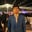Related Tags

cryptography

# Properties of a cryptographically secure and useful hash functionAhsan Amin

### What is a hash function?

It is a function that takes arbitrary-sized data and maps it to a fixed-size integer value.

Working on a hash function

### Uses of hash functions

Some uses cases of hash functions in cryptography are as follows:

• Digital signatures: To sign a message, we first take a cryptographic hash of the message and then encrypt it with the receiver's public key.
• Storing passwords in a database: Hashes of passwords are used instead of storing in plain text so that even if the database is compromised, the hacker cannot get access to the user's passwords as cryptographic hash functions are pre-image resistant.
• Data integrity: Take a hash of all our files before leaving a system and then recompute the hash to check if the files have been changed or not.

### Properties of cryptographic hash functions

Properties of good cryptographic hash functions are as follows:

#### Collision resistant

It is a property of a hash function h for which it is computationally very hard to find two distinct inputs, A and B, for which h(A) = h(B).

#### Pre-image resistance

It is computationally infeasible to invert a hash function (find an input that hashes to the given output). The following is not possible.

A hash function cannot be reverse-engineered

#### Second pre-image resistance

It is computationally infeasible to find a second input that hashes to the same output as the given input. e.g., Given h(Cat) = AB38DA, it is computationally very hard to find another input that maps to the same output.

We cannot find another input that has the same output hash

#### Large output space

Outputs of cryptographic hash functions usually are very large integers (represented in binary as bits). The output of the SHA-256SHA-256 belongs to a family of hash algorithms known as Secure Hash Algorithm 2 hash function is 256 bits long, and the total number of possible outcomes is $2^{256} = 1.1579209 \times 10^{77}.$ Thus, using a brute force approach on this number of outputs will take years to compute, thus making it ineffective and our hash function more secure.

#### Deterministic

A given input x to a hash function H will always generate the same output.

#### Avalanche effect

A small change in the input will result in a completely different output hash.

#### Puzzle friendliness

A hash function is puzzle friendly if no solution exists, which is better than just making random guesses. Thus, the only way to find a solution is the brute force which takes $2^{n}$, where n = number of bits in the output to guess the correct answer.

#### Fixed-length mapping

The output size of a hash function is fixed and independent of the input size. The output size of the SHA-256 hash function is 256 bits for any arbitrary-sized input.

RELATED TAGS

cryptography

CONTRIBUTORAhsan Amin
RELATED COURSES

View all Courses

Keep Exploring

Learn in-demand tech skills in half the time